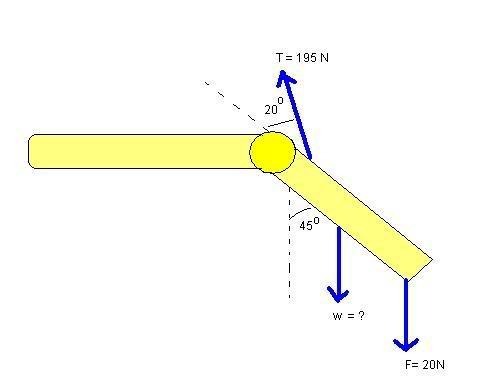# Equilibrium and Torque of quadricep muscles

• bortron
I've tried re-working the problem a few different ways, and I'm still not sure where I went wrong. In the end, I think it may have been that I wasn't including the length of the leg in my calculations, which would lead to the incorrect answer.f

## Homework Statement

The large quadricep muscles in the upper leg terminate at the lower end in a tendon attached to the upper end of the tibia. The forces on the lower leg are defined as: T is the tension in the tendon, w is the force of gravity acting on the lower leg, and F is the weight of the foot.
Find w when the tendon is at an angle of 20 degrees with the tibia, assuming T= 195 N, F= 20 N and the leg is extended at an angle of 45 degrees with the vertical. Assume that the center of gravity of the lower leg is at its center and that the tendon attaches to the lower leg at a point one fourth of the way down the leg. Include a diagram of the situation.

## Homework Equations

In equilibrium: Torque ccw = - Torque cw

## The Attempt at a Solution

The way I see this, you set the sums of opposing torques equal to one another and solve for w, so:

*(Torque from tendon) = (Torque from weight of lower leg) + (Torque from weight of the foot)

*r T sin 20 = r w sin 45 + r F sin 45

*(.25)(195)(.342) = (.5)(w)(.707) + (1)(20)(.707)

*w = 7.16 N

This seems wrong to me because it would mean that the mass of the lower leg was much less than the mass of the foot, which is unlikely in the real world. So where have I gone wrong?
The length of the leg is not given, but I think if you just set "r" equal to the length of the leg multiplied by the force's relative distance from the pivot, the length of the leg cancels allowing the use of just the relative distances (that's where I get the .25, .5, and 1 used above).
I used the sin of the angle between the force an the pivot, given as 20 for the tendon and 45 for w and F. (at least this is how I interpret what the problem is saying, any other interpretations?)

Any help would be very greatly appreciated, thanks so much.

Here is the diagram.

Here is the diagram. =)

URL: http://img.photobucket.com/albums/v22/spacezombee/legtorque.jpgThanks for the help!

if your diagram is correct... you are correct.

Well maybe that's it then...

That diagram was supplied with the problem... so perhaps that is the correct answer.

I noticed that a version of this same problem that asked the student to solve for T with slightly different numbers appears elsewhere on the internet, so possibly my professor just came in and changed the numbers without realizing it made the answer make no sense.

Thanks for the responses!